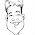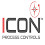## Sunday, 29 May 2016

by Giovanni Carrera, rev. 28/05/16
A voltage to frequency converter can realize an opto-isolated analog input for Arduino or other microcontroller systems. This circuit is particularly suitable for industrial control plants with 4-20mA sensors.

### Introduction

The signals from field sensors can be affected by noise generated by power surges, lightning strikes or other EMI (Electromagnetic Interference) sources and also by ground potential differences. One method to avoid most of these problems is to use a complete isolation from the field.
The isolation of an input sensor will require a separate power supply to power the field device and the circuit that realize the insulation itself.
In the 80s, when the microcontrollers hadn’t  digital to analog converters (ADC) integrated, I had designed a system with voltage to frequency converters to achieve high-resolution analog inputs and an easy true isolation with the field sensors.  Only drawback was the low sampling rate, but usually high frequencies aren’t required in industrial plants.
The following figure shows a three channels board that I designed many years ago for a 6502 control system.

Figure 1 - A three analog isolated channels VFC board

### The circuit

So I think up to use this circuit also with Arduino. The diagram of Figure 2 shows the circuit that accepts an input voltage from about 20mV to 5V or a current of 4 to 20 mA (with the jumper W1 inserted). The two resistors in parallel R2 and R3 give a value of about 250 ohms, in order to have 1V to 5V for 4mA to 20mA input.
Just three wires and a resistor are required to connect the circuit to the Arduino Uno. The output of the opto-coupler should be connected to the digital input D5 with a pull-up 2.2kW resistor connected to the +5V of Arduino.
Figure 2 - Schematic of the circuit.
If an input range of 10V is required, a 15V power supply is necessary, so you have to change the 7808 regulator with a 7815.
The resistor R10 reduces the switching time of the phototransistor.
The circuit of the converter is obtained from the datasheet of the LM331.
The output frequency is:
Fout = Vin*(R6+P1)/(2.09*R4*R7*C3)
This circuit, with the values used,  has a conversion factor of about 1kHz/V.
The duration of output pulses is:
T = 1.1* R7*C3 = 74.8 [ms]

### Hardware components

1x Arduino board

Passive components

 R1= 100kW ±1% metal film R2= 3.3kW ±1% metal film R3= 270W ±1% metal film R4= 100kW ±1% metal film R5= 47W ±5% R6= 12kW ±1% metal film R7= 6.8kW ±1% metal film R8= 10kW ±5% R9= 330W ±1% 1/4W R10= 220kW ±5% P1= 5kW multiturn C1= 100nF Mylar C2= 1MF Mylar C3= 10nF Mylar C4 = 100nF ceramic AVX C5 = 4.7 MF Electrolytic C6= 220 MF Electrolytic T1=230V (or 115V), 12V, 1VA transformer

Active components
 U1= LM331 U2= LM7808 Pd1= 4x1N4001 OP1=4N25

### Calibration and measurements analysis

A very stable power supply, as source, a frequency counter and an oscilloscope are been used for testing the circuit.
The 13 measurements were analyzed with a spreadsheet using a linear regression and the results were very good, as you can see from the figure below graph.
Figure 3 – Calibration curve of the circuit.
As you can see from the formula of the regression line, the correlation coefficient is equal to one, using six decimal digits.

### Arduino interface and program

Arduino can measure the frequency in two ways:
·        period measurement, using the pulsein() function;
·        frequency measurement, with CPU Timer/Counters , using special libraries.
The first method uses twice the function pulsein() in order to measure the HIGH time and LOW time of the signal, with a microsecond resolution. The sum of the two measurements is the period of the signal. For a 5kHz signal, the period is 200 µs = HIGH time + LOW time = 125+75 µs. The time resolution is  relatively low and the accuracy of measurement is also affected by the time of the program instructions.
The positive aspect about this method is the measurement speed which is slightly higher than the measured period. At a disadvantage, in addition to the less accuracy, it is most sensitive to the electrical grid noise (50 or 60Hz).
For these reasons, I prefer a frequency measurement with respect to the period. The measurement time is higher but you get a higher precision and precise sampling times. In addition, choosing a measurement period multiple of that of the electrical grid, it has excellent noise immunity.
I use the FreqCounter library by Martin Nawrath KHM LAB3:
This library uses Timer/Counter1 for counting pulses on rising edge of T1/PD5/digitalPin5 and Timer/Counter2 for the gate-time generation with 1 ms interrupts.
I chose a gate time equal to 1000 ms to mediate the count on a period of 50 or 60 mains cycles. In this case you get a resolution that is five times greater than that of Arduino Uno.
The Arduino sketch of the following example is very simple:

#include <FreqCounter.h>

void setup() {
Serial.begin(9600);  // connect to the serial port
}

long int frq;
void loop() {

FreqCounter::f_comp= 0;   // Set compensation to 0
FreqCounter::start(1000);  // Start counting with gatetime of 1000ms

Serial.println(frq);       // print result
delay(100);
}

By comparing the frequency meter readings with the values transmitted by Arduino I found only small differences and the error is very good, as shown in the following table.
 Frequency Meter [Hz] Arduino FreqCounter [Hz] Error % 105 104 -0.952% 1009 1010 0.099% 1995 1998 0.150% 3164 3167 0.095% 3993 3997 0.100% 4964 4969 0.101%
With appropriate libraries and with other CPU, you can have multiple frequency inputs and then various isolated analog channels.

References
1.      “LMx31x Precision Voltage-to-Frequency Converters”, Texas Instruments, SNOSBI2C –JUNE 1999–REVISED SEPTEMBER 2015.

2.      “Arduino Frequency Counter Library”, Laboratory for Experimental Computer Science at the Academy of Media Arts Cologne, http://interface.khm.de/index.php/lab/interfaces-advanced/arduino-frequency-counter-library/

1.2.3.